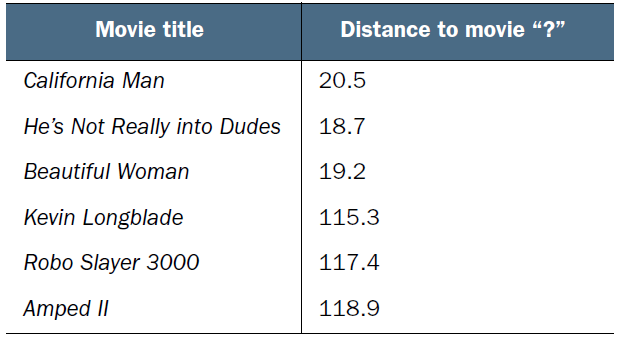kNN

$(x_1, y_1)$
$(x_2, y_2)$

$d=\sqrt{(x_1-x_2)^2+(y_1-y_2)}$$d=\sqrt{(18-3)^2+(90-104)^2}\approx 20.518$1
import numpy as np
2
3
4
class Movie:
5
def __init__(self, kicks, kisses, type, name=""):
6
# type为1表示爱情电影，为2表示动作电影
7
self.name = name
8
self.kicks = kicks
9
self.kisses = kisses
10
self.type = type
11
12
13
class kNN:
14
def __init__(self, k):
15
self.k = k
16
self.train_set = []
17
18
def feed(self, kicks, kisses, type):
19
self.train_set.append([0, kicks, kisses, type])
20
21
def predict(self, kicks, kisses):
22
for i in range(len(self.train_set)):
23
self.train_set[i] = np.sqrt((kicks - self.train_set[i]) ** 2 +
24
(kisses - self.train_set[i]) ** 2)
25
self.train_set.sort()
26
27
for i in range(self.k):
28
29
30
else:
31
32
max = 0
33
max_type = -1
34
35
36
37
max_type = k
38
return max_type
39
40
41
if __name__ == "__main__":
42
# k为3的kNN算法
43
predictor = kNN(3)
44
# 从文件中读取训练集
45
with open('F:\\Desktop\\test.txt', 'r') as file:
46
47
for i in range(len(content)):
48
info = content[i].split('\t')
49
predictor.feed(int(info), int(info), int(info))
50
# 从控制台读取亟待预测的数据
51
kick = int(input("Please type count of kicks within this movie: "))
52
kiss = int(input("Please type count of kisses within this movie: "))
53
if predictor.predict(kick, kiss) == 1:
54
print("The result of the predicted type of this movie is ", "Romance")
55
else:
56
print("The result of the predicted type of this movie is ", "Action")
Copied!